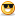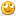# Thread: LED's and Resistors Guide

1. ##Re: LED's and Resistors Guide

Seems like you never learned the electrical basics. Short explanation :

Series : The most basic circuit.If you try to make a circuit like that running on for example 6volts AC with 3 10v light bulbs, you will notice that the light bulbs will not glow very much. And if you remove a light (or if it burns) the circuit is cutted, closed, no more current is passing.

Parallel : ''Safer'' circuit, a bit more complicated but it is logical.Here, the power is equal everywhere, meaning that if the circuit is running 5V the current will be of 5V everywhere. You will probly have to use one resistor for each LEDs if you are not using the same leds in the circuit, or one big resistor for the lot; but don'T trust me for resistors, I dont know how they works, and how to use them. So as you have read in the first post formulas ^^^^ each LED will be using its own voltage and amps settings. Basically, 3 6V bulbs made for 10V will light at 6V full efficiency (like if you would only be using one 6V bulb on series). But this circuit can be a bit more dangerous, if you happen to make a shortcircuit error (like removing a light and just tie the wires together), if you ahve nothing on the circuit that will eat a bit of power then itll shorten.

I sucks for explanations, but its about that for series and parallel. Just tell me if I said something wrong, but its hard to tell everything in english (im originally french, and still not perfecly bilingual).

Hastly said :
Series uses less power, but the lights wont show up as brighly as if they were linked in parallel. Parallel allows your leds to bright as they would normally, and the circuit won't close if one LED closes.Reply With Quote

2. ##Re: LED's and Resistors Guide

uh.. i know.. thanks.. nice diagrams.

This would be the equation for parallel.

Example:

three 3.6 leds with a mA of 20 on a 5 volt rail.

In parallel you multiply the mA by the number of Led's you want to use so since we want 3 leds it would be a mA of 60.

(5 volt[power] - 3.6[led]) / 0.06 amps [60 mA]
= 23

do i put a 23ohm resistor 3 times in (once for each bulb) or 1 time, resisting for all 3?Reply With Quote

3. ##Re: LED's and Resistors Guide

Once should be fine on paper; though in real life, there may be other factors. Watch for xmastree catching this thread, he seems to know his stuff, corrected me plenty of times. (although he's not the only one who knows his stuff, don't take that the wrong way...)

-DaveReply With Quote

4. ##Re: LED's and Resistors Guide

ah, cool. i am thinking about making a UV flashlight since they cost so much to buy.. and stick some in my case.Reply With Quote

5. ##Re: LED's and Resistors GuideOriginally Posted by DaveW
Watch for xmastree catching this thread, he seems to know his stuff,
How could I refuse?Sorry I'm late, been at a Rotary convention all weekend.Originally Posted by Nagoshi
And if you remove a light (or if it burns) the circuit is cutted, closed, no more current is passing.
The circuit will be open. Open circuit passes no current.Originally Posted by Nagoshi
Parallel :
Here, the power is equal everywhere, meaning that if the circuit is running 5V the current will be of 5V everywhere.
I know what you're trying to say, but that's a little misleading. The voltage across each bulb will be the same as the battery voltage. In the series circuit, assuming identical bulbs, they will share the battery voltage equally. In each case, the power will be equal.

As for 3x3.6V LEDs from a 5V source, better to give them individual resistors. firstly, because they might not be exactly the same, and the one with the lowest forward voltage will draw more current than the others, and secondly because if one dies, and stops conducting, it will force a higher current through the remaining ones.Originally Posted by humanentropy
LEDS in series is more efficient since the load on the power supply is the same as for one. However, the subsequent lower value for the series resistor makes it more sensitive to voltage fluctuations.

Why? Well the voltage across the resistor will be lower, so a shift of, say 0.5V is a higher proportion of the resistor's voltage, so it will have a greater effect on the current.

Example... nominal 12V, 3.6V LED, 10mA required.

For one LED, the resistor would be 840 ohms, with 8,4V across it @ 10mA
Three LEDs, the resistor would be 120 ohms, with 1.2V across it @10 mA

At 11.5V, the one LED will have 9.4mA, the three will have 5.8mA
At 12.5V, the one LED will have 10.5mA, the three will have 14.2mA

+/- 0.5V has a much greater effect on 1.2V than it does on 8.4V, so the higher the voltage across the resistor, the better the regulation.

Getting complicated?For three 3.6V LEDs in a computer, I would give them each a resistor and run them off the 12V rail.Reply With Quote

6. ##Re: LED's and Resistors Guide

For one LED, the resistor would be 840 ohms, with 8,4V across it @ 10mA
Three LEDs, the resistor would be 120 ohms, with 1.2V across it @10 mA

so if i were in parallel, should i give each of the three LEDs a resistor, i should put put an 840 ohm resistor for each LED, right?

if i were in parallel, should i use one resistor for all three LEDs, i should use a 120ohm resistor before splitting off, right?Reply With Quote

7. ##Re: LED's and Resistors GuideOriginally Posted by humanentropy
so if i were in parallel, should i give each of the three LEDs a resistor, i should put put an 840 ohm resistor for each LED, right?
Yes'
if i were in parallel, should i use one resistor for all three LEDs, i should use a 120ohm resistor before splitting off, right?
No, the 120 example is if they are in series. If you put them directly in parallel and use one resistor (not a good idea) it would need to be 280ohms.Reply With Quote

8. ##Re: LED's and Resistors Guide

ah, i meant series for the second, but now i understand!

thanks a bundle. now to look for good led websites..Reply With Quote

9. ##Re: LED's and Resistors GuideOriginally Posted by humanentropy
now to look for good led websites..
http://ledmuseum.home.att.net/Reply With Quote

10. ##Re: LED's and Resistors GuideOriginally Posted by xmastree
http://www.hebeiltd.com.cn/?p=leds.5mm i cant find the current rating.

http://www.123leds.com/ i might use thatReply With Quote

####Posting Permissions

• You may not post new threads
• You may not post replies
• You may not post attachments
• You may not edit your posts
•